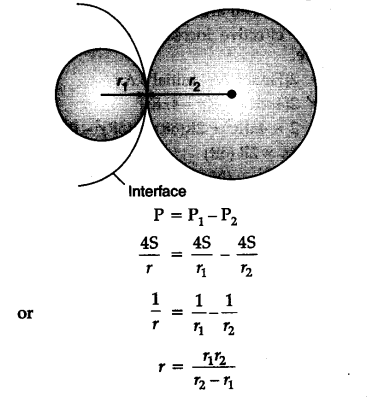# Derive an expression for the radius of interference when two soap bubbles of different radii are in contact

Consider two soap bubbles of radii r1 and r2 in contact with each other as shown in figure.
Let r be the radius of the common boundary. If Px and P2 are the excess pressure on the two sides of one interface then the resultant excess pressure